Tutorials
machine learning

LDA2vec: Word Embeddings in Topic Models

Learn more about LDA2vec, a model that learns dense word vectors jointly with Dirichlet-distributed latent document-level mixtures of topic vectors.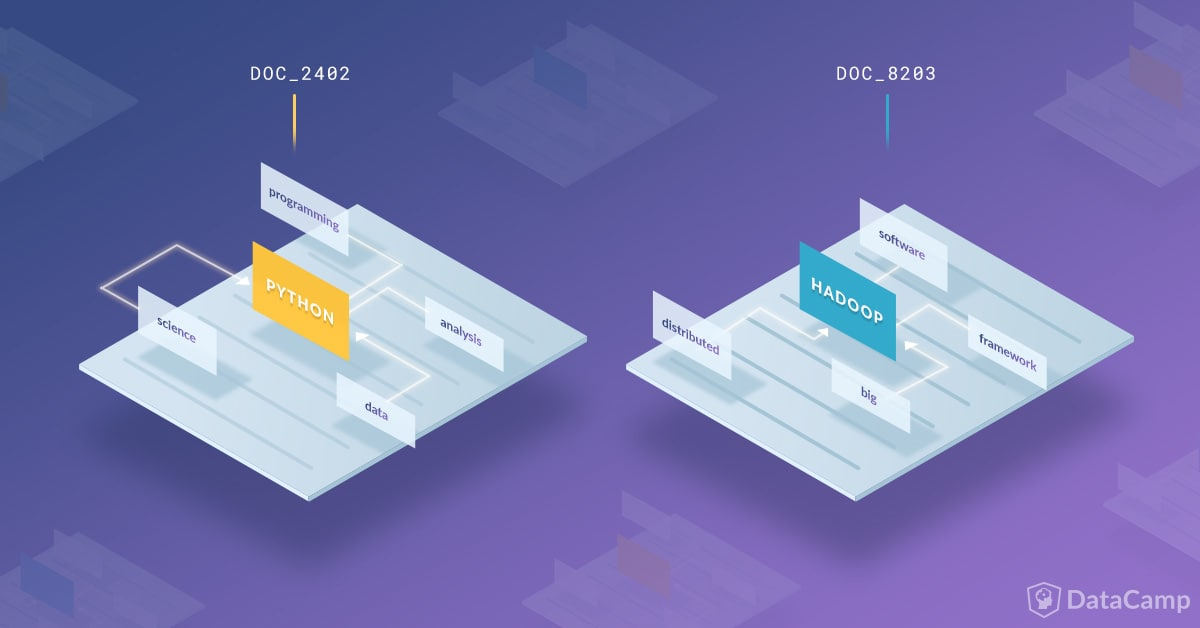This blog post will give you an introduction to lda2vec, a topic model published by Chris Moody in 2016. lda2vec expands the word2vec model, described by Mikolov et al. in 2013, with topic and document vectors and incorporates ideas from both word embedding and topic models.

The general goal of a topic model is to produce interpretable document representations which can be used to discover the topics or structure in a collection of unlabelled documents. An example of such an interpretable document representation is: document X is 20% topic a, 40% topic b and 40% topic c.

Today's post will start off by introducing Latent Dirichlet Allocation (LDA). LDA is a probabilistic topic model and it treats documents as a bag-of-words, so you're going to explore the advantages and disadvantages of this approach first.

On the other hand, lda2vec builds document representations on top of word embeddings. You'll learn more about word embeddings and why they are currently the preferred building block in natural language processing (NLP) models.

Latent Dirichlet Allocation: Introduction

A topic model takes a collection of unlabelled documents and attempts to find the structure or topics in this collection. Note that topic models often assume that word usage is correlated with topic occurence. You could, for example, provide a topic model with a set of news articles and the topic model will divide the documents in a number of clusters according to word usage.

Topic models are a great way to automatically explore and structure a large set of documents: they group or cluster documents based on the words that occur in them. As documents on similar topics tend to use a similar sub-vocabulary, the resulting clusters of documents can be interpreted as discussing different 'topics'.

Latent Dirichlet Allocation (LDA) is an example of a probabilistic topic model. What this exactly means, you'll learn in the following sections: you'll first come to understand how LDA starts from a bag-of-words description to represent the different documents. Then, you'll see how these representations are used to find the structure in the document collection.

Bag-of-words

Traditionally, text documents are represented in NLP as a bag-of-words.

This means that each document is represented as a fixed-length vector with length equal to the vocabulary size. Each dimension of this vector corresponds to the count or occurrence of a word in a document. Being able to reduce variable-length documents to fixed-length vectors makes them more amenable for use with a large variety of machine learning (ML) models and tasks (clustering, classification, ...).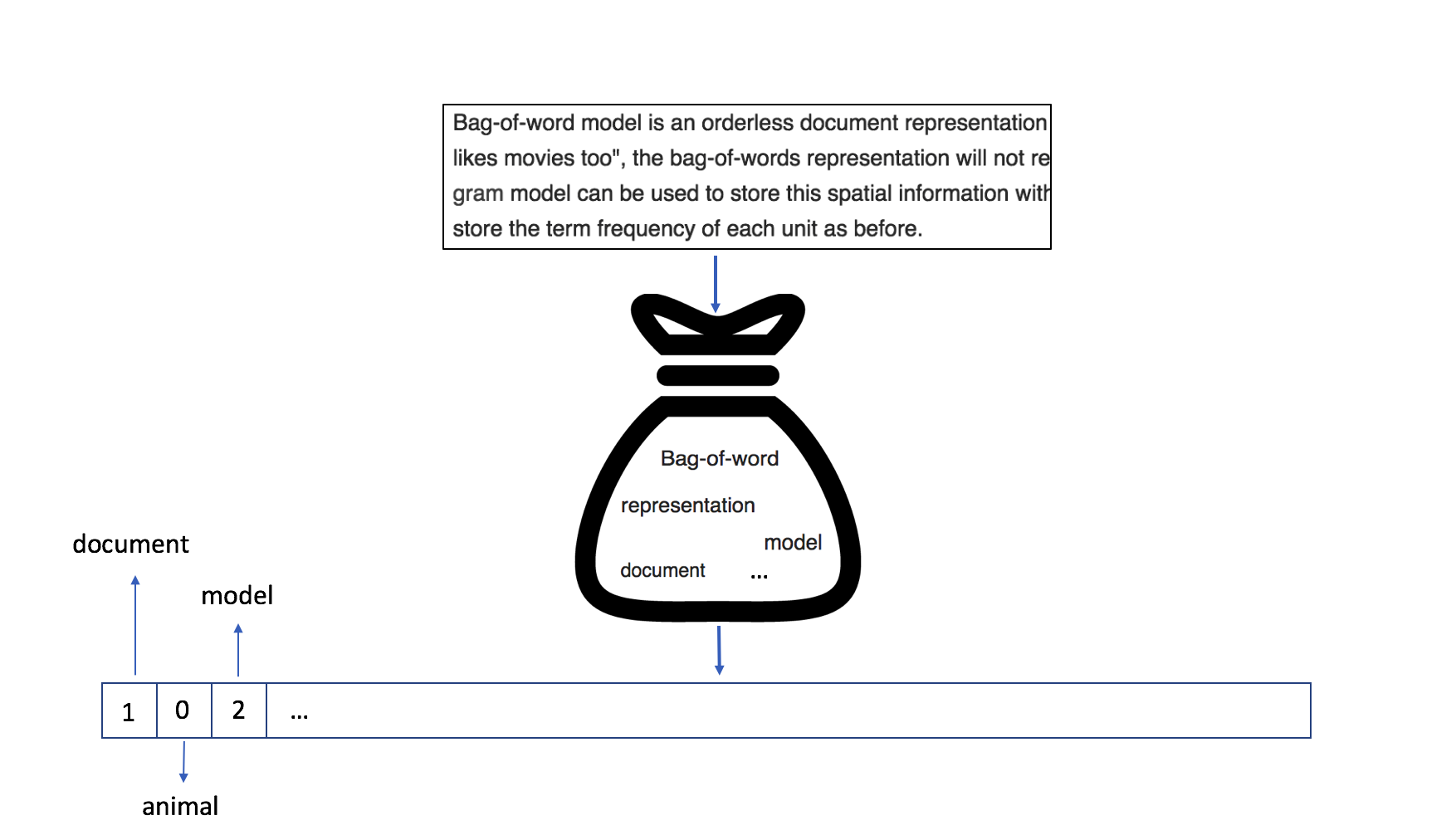The image above illustrates how a document is represented in a bag-of-word model: the word "document" has a count of 1, while the word "model" occurs twice in the text.

Although the bag-of-words results in a sparse and high-dimensional document representation, good results on topic classification are often obtained if a lot of data is available. You can always read up on the recent Facebook paper on topic classification.

A fixed-length document representation means you can easily feed documents with varying length into ML models (SVM's, k-NN, Random Forests, ...). This allows you to perform clustering or topic classification on documents. The structural information of the document is removed and models have to discover which vector dimensions are semantically similar. Mapping for example ‘feline’ and ‘cat’ on different dimensions is less intuitive, as the model is forced to learn the correlation between these different dimensions.

The LDA model

When training an LDA model, you start with a collection of documents and each of these is represented by a fixed-length vector (bag-of-words). LDA is a general Machine Learning (ML) technique, which means that it can also be used for other unsupervised ML problems where the input is a collection of fixed-length vectors and the goal is to explore the structure of this data.

To implement an LDA model, you first start by defining the number of 'topics' that are present in your collection of documents. This sounds straight-forward, but is often less intuitive than it sounds if you are working with vast amounts of documents.

Training an LDA model on $$N$$ documents with $$M$$ topics corresponds with finding the document and topic vectors that best explain the data.

Note that this tutorial will not cover the full theory behind LDA in detail (see this paper by Blei et al. for that), as the focus is more getting the general idea across.

Assume that the vocabulary in the documents consists of $$V$$ words.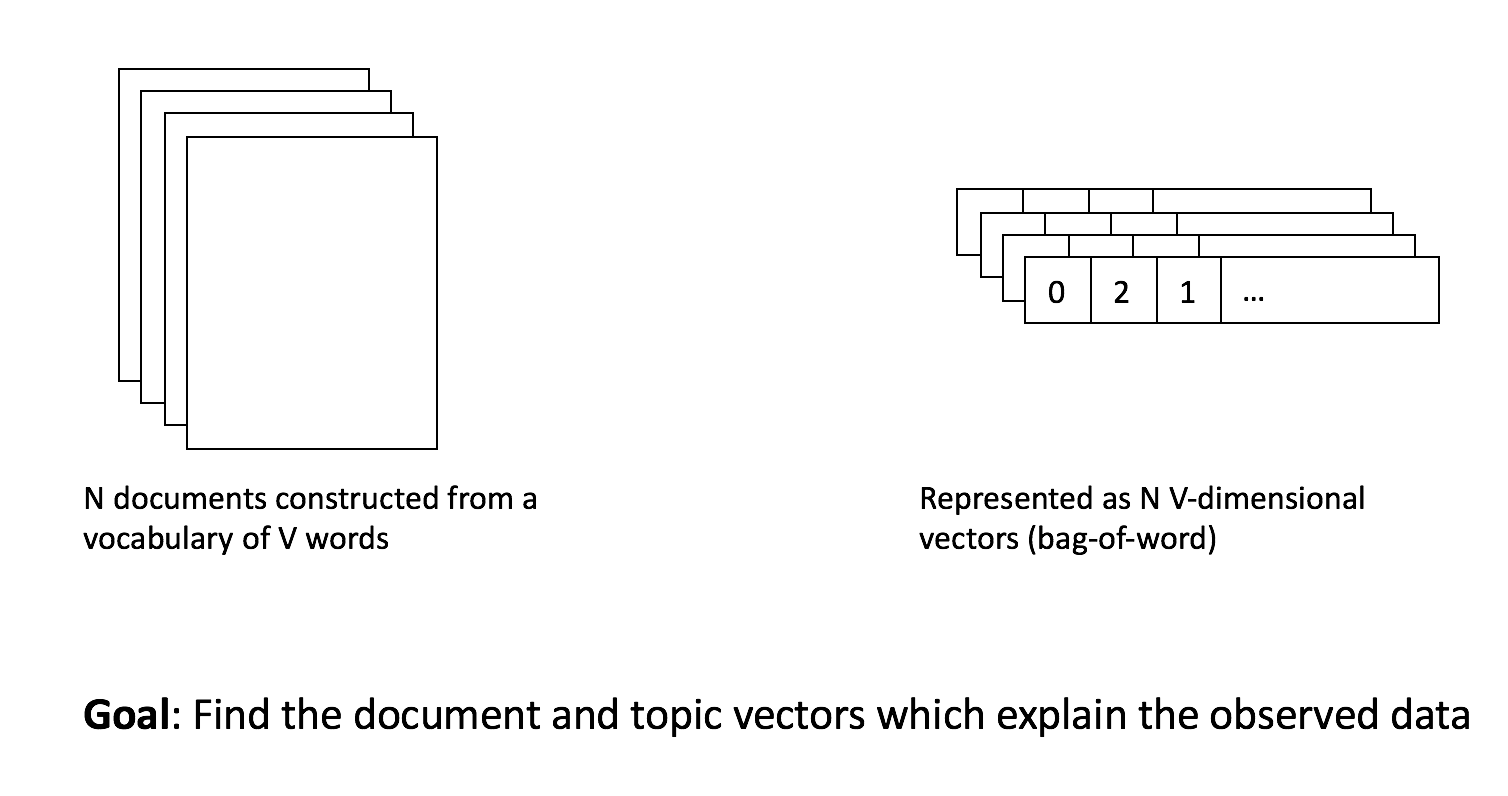Each of the $$N$$ documents wil be represented in the LDA model by a vector of length $$M$$ that details which topics occur in that document. A document can consist of 75% being 'topic 1' and 25% being 'topic 2'. Often, LDA results in document vectors with a lot of zeros, which means that there are only a limited number of topics occur per document. This corresponds with the idea that documents typically only talk about a limited number of topics. This significantly improves the human interpretability of these document vectors.

Each of the $$M$$ topics is represented by a vector of length $$V$$ that details which words are likely to occur, given a document on that topic. So for topic 1, 'learning', 'modelling' and 'statistics' might be some of the most common words. This means that you could then say that this is the 'data science' topic. For topic 2, the words 'GPU', 'compute' and 'storage' could be the most common words. You could interpret this as the 'computing' topic.

The following image illustrates the LDA model visually. The goal of the model is to find the topic and document vectors that explain the original bag-of-word representation of the different documents.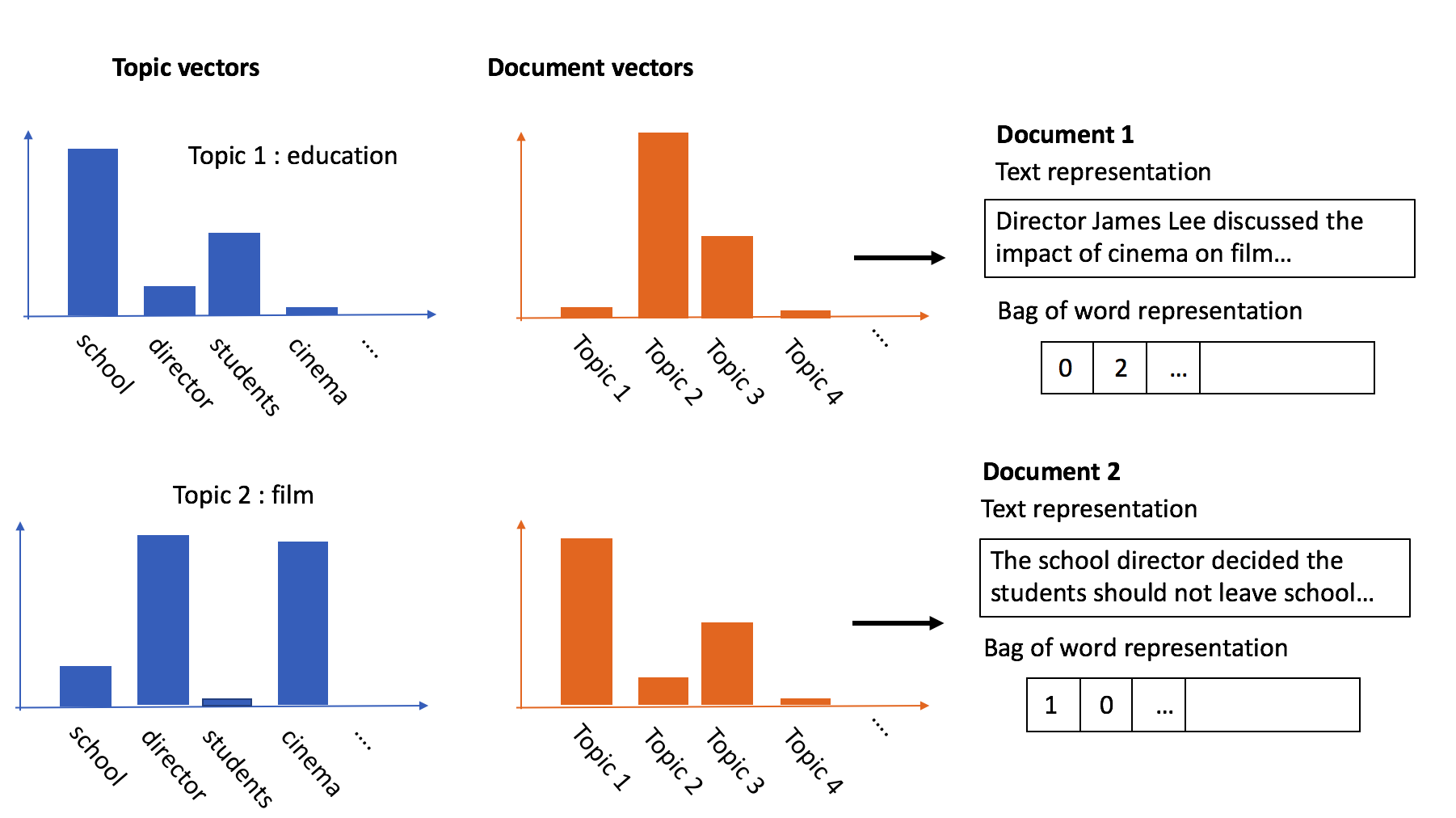It is important to notice that you are relying on the assumption that the topic vectors will be interpretable, otherwise the output of the model is pretty much garbage. Essentially, you are assuming that the model, given enough data, will figure out which words tend to co-occur, and will cluster them into distinct 'topics'.

LDA is a simple probabilistic model that tends to work pretty good. The document vectors are often sparse, low-dimensional and highly interpretable, highlighting the pattern and structure in documents. You have to determine a good estimate of the number of topics that occur in the collection of the documents. In addition, you have to manually assign a distinct nominator/‘topic’ to the different topic vector. As a bag-of-words model is used to represent the documents, LDA can suffer from the same disadvantages as the bag-of-words model. The LDA model learns a document vector that predicts words inside of that document while disregarding any structure or how these words interact on a local level.

Word Embeddings

One of the problems of the bag-of-words representation is that the model is responsible for figuring out which dimensions in the document vectors are semantically related. One might imagine that leveraging information on how words are semantically correlated to each other will improve a model’s performance and this is exactly what word embeddings promise.

With word embeddings, words are represented as fixed-length vectors or embeddings. Several different models exist to construct embeddings, but they are all based on the distributional hypothesis. That means that "a word is characterised by the company it keeps".

The goal of word embeddings is to capture semantic and syntactic regularities in language from large unsupervised sets of documents, such as Wikipedia. Words that occur in the same context are represented by vectors in close proximity to each other.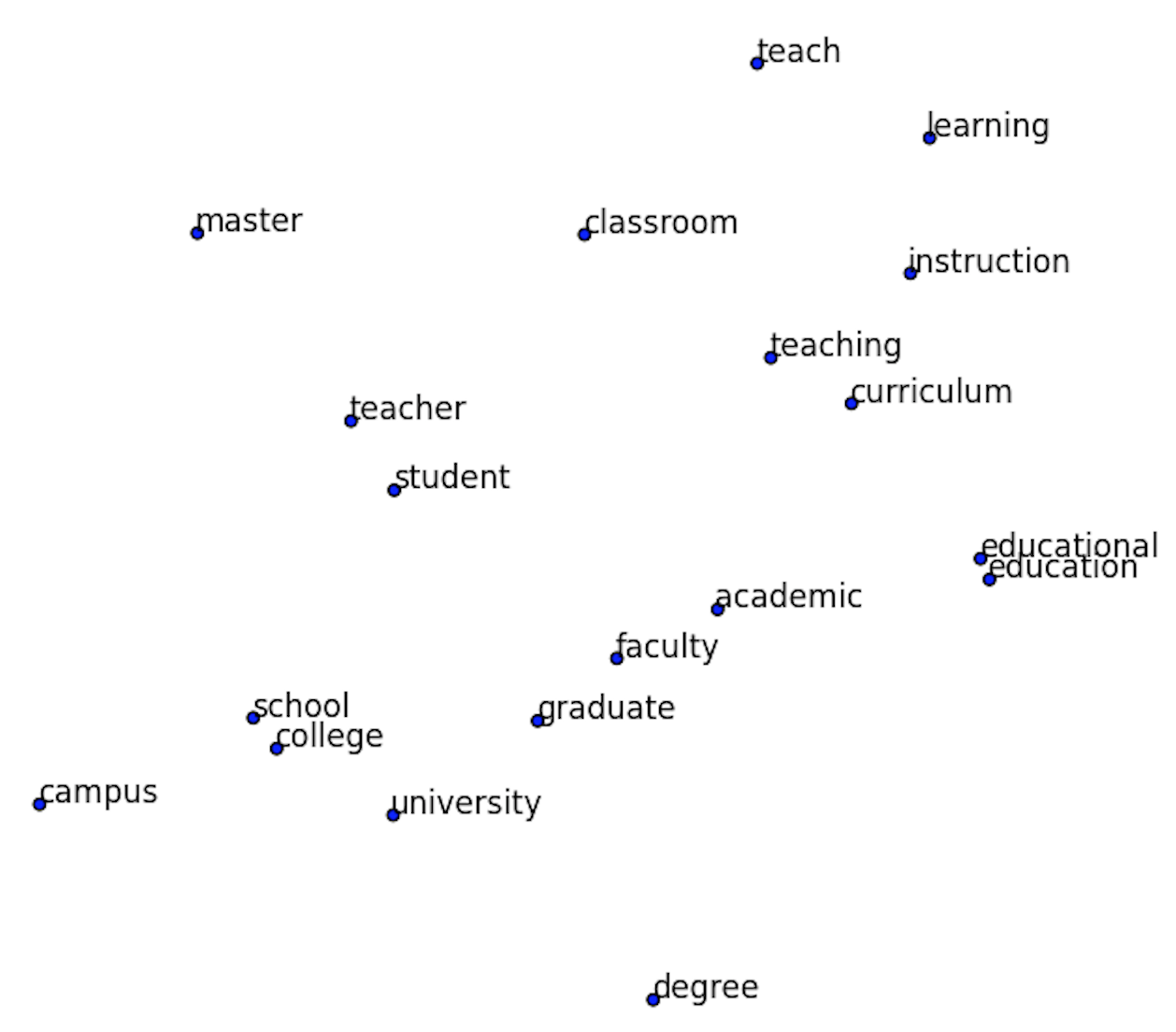Image taken from "Visualizing Word Embeddings with t-SNE"

The image above is a projection of the word embedding space to 2D space using t-Distributed Stochastic Neighbor Embedding (t-SNE). t-SNE is a dimensionality reduction method that you can use to visualise high-dimensional data. The method takes the word embeddings as input, and projects them onto two-dimensional space which can be easily visualised in a plot. Only a subsection of the word space is investigated, focussing on words close to 'teacher’. Instead of representing words by uninformative dimensions in a vector, words can be represented by semantically correlated vectors using word embeddings.

When using word embeddings, an ML model can leverage information from a large a collection of documents, also known as a "corpus", by embedding it in the vector representations. This is not possible with bag-of-words models, which can hurt model performance when not a lot of data is available. Word embeddings lead to document representations that are not fixed-length anymore. Instead, documents are represented by a variable-length sequence of word vector embeddings. While some deep learning techniques, such as Long Short-Term Memory (LSTM)'s, convolutional nets with adaptive pooling, ..., are able to deal with variable length sequences, a lot of data is often necessary to properly train them.

word2vec

As you read in the introduction, word2vec is highly popular word embedding model, developed by Mikolov et al. Note that several other word embedding models exist within the field of distributional semantics. Although several tricks are required to obtain high-quality word embeddings, this tutorial will only focus on the core idea behind word2vec.

The following training procedure is used in word2vec to obtain the word embeddings.

1. Select a (pivot) word in the text. The context words of the current pivot word are the words that occur around the pivot word. This means that you're working within a fixed-length window of words. The combinations of the pivot and context words constitute a set of word-context pairs. The image below was taken from the Chris Moody's blog on lda2vec. In this text fragment, 'awesome' is the pivot word and the words around it are taken as context words, resulting in 7 word-context pairs.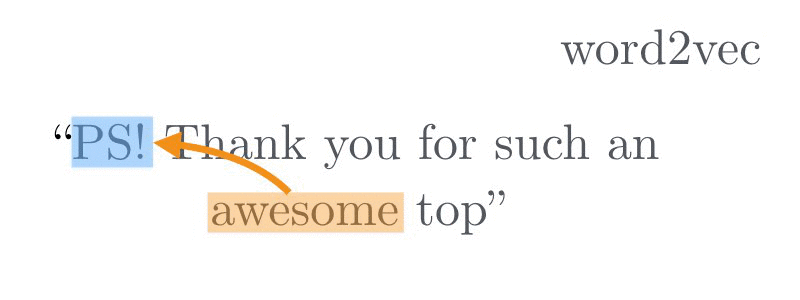Image taken from "Introducing our Hybrid lda2vec Algorithm"
2. Two variants of the word2vec model exist: a. In the bag-of-words architecture (CBOW) the pivot word is predicted based on a set of surrounding context words (i.e. given 'thank', 'such', 'you', 'top' the model has to predict 'awesome'). This is called a bag-of-words architecture as the order of context words does not matter. b. In the skip-gram architecture, the pivot word is used to predict the surrounding context words (i.e. given 'awesome' predict 'thank', 'such', 'you', 'top' ). The following image depicts the two different word2vec architectures. Note that a relatively simple (two layer) neural model is used (compared to deep neural models in computer vision).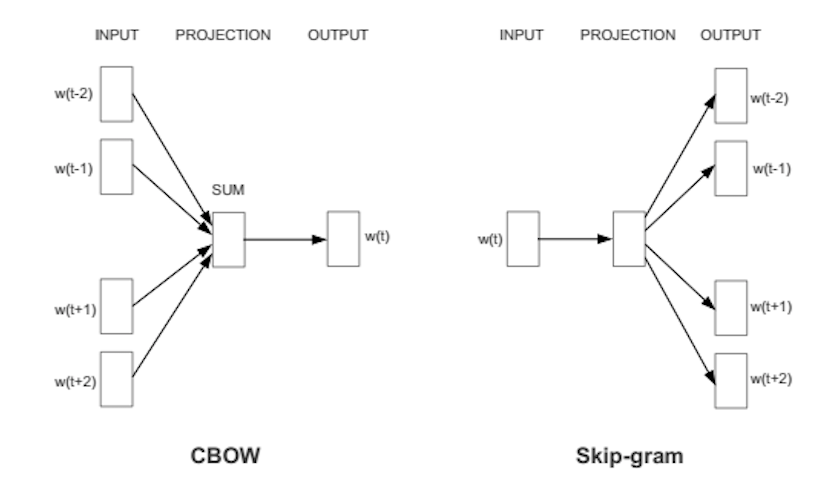Image taken from "Efficient Estimation of Word Representations in Vector Space" (Mikolov et al., 2013)

By training the model on a large corpus, you will obtain word embeddings (the weights in the projection layer) that encode semantical information as well as some interesting properties: it is possible to perform vector arithmetic, such as $$king - man + woman = queen$$.

Word vectors are a useful representation compared to, for example, a simple one-hot encoded representation. They allow to encode statistical information from a large corpus into other models, such as topic classification or dialogue systems. The word vectors are often dense, high-dimensional and uninterpretable. Consider the following example: [ -0.65, -1.223, ..., -0.252, +3.2]. While in LDA dimensions correspond approximately to topics, this is typically not the case for word vectors. Each word is assigned a context-independent word vector. The semantic meaning of words is, however, highly dependent on context. The word2vec model learns a word vector that predicts context words across different documents. As a result, document-specific information is mixed together in the word embeddings.

lda2vec

Inspired by Latent Dirichlet Allocation (LDA), the word2vec model is expanded to simultaneously learn word, document and topic vectors.

Lda2vec is obtained by modifying the skip-gram word2vec variant. In the original skip-gram method, the model is trained to predict context words based on a pivot word. In lda2vec, the pivot word vector and a document vector are added to obtain a context vector. This context vector is then used to predict context words.

In the next section, you will see how these document vectors are constructed and how they can be used similarly as document vectors in LDA.

lda2vec Architecture

The idea of integrating context vectors in the word2vec model is not a new idea. Paragraph vectors, for example, also explored this idea in order to learn fixed-length representations of variable-length text fragments. In their work, for each text fragment (size of a paragraph) a dense vector representation is learned, similar to the learned word vectors.

The downside of this approach is that the context/paragraph vectors resemble typical word vectors, making them less interpretable as, for example, the output of LDA.

The lda2vec model goes one step beyond the paragraph vector approach by working with document-sized text fragments and decomposing the document vectors into two different components. In the same spirit as the LDA model, a document vector is decomposed into a document weight vector and a topic matrix. The document weight vector represents the percentage of the different topics, whereas the topic matrix consists of the different topic vectors. A context vector is thus constructed by combining the different topic vectors that occur in a document.

Consider the following example: in the original word2vec model, if the pivot word is 'French', then possible context words might be 'German', 'Dutch', 'English'. Without any global (document-related) information, these would be the most plausible guesses.

By providing an additional context vector in the lda2vec model, it is possible to make better guesses of context words.

If the document vector is a combination of the ‘food’ and ‘drinks’ topics, then 'baguette', 'cheese' and 'wine' might be more suitable. If the document vector is similar to the 'city' and ‘geography’ topics, then 'Paris', 'Lyon' and 'Grenoble' might be more suitable.

Note that these topic vectors are learned in word space, which allows for easy interpretation: you simply look at the word vectors that are closest to the topic vectors. In addition, constraints are put on the document weight vectors, to obtain a sparse vector (similar to LDA), instead of a dense vector. This enables easy interpretation of the topic content of different documents.

In short, the end-result of the lda2vec is a set of sparse document weight vectors, as well as easily interpretable topic vectors.

Although the performance tends to be similar to traditional LDA, using automatic differentiation methods makes the method scalable to very vast datasets. In addition, by combining the context vector and word vector, you obtain ‘specialised’ word vectors, which can be used in other models (and might outperform more ‘generic’ word vectors).

lda2vec Libraries

Lda2vec is a fairly new and specialised NLP technique. As it builds on existing methods, any word2vec implementation could be extended into lda2vec. Chris Moody implemented the method in Chainer, but other automatic differentiation frameworks could also be used (CNTK, Theano, ...). A Tensorflow implementation was also made publicly available.

An overview of the lda2vec Python module can be found here. As training lda2vec can be computationally intensive, GPU support is recommended for larger corpora. In addition, in order to speed up training, the different word vectors are often initialised with pre-trained word2vec vectors.

Finally, lda2vec was discussed as a topic model, but the idea of adding context vectors to the word2vec model can also be defined more generally. Consider for example documents written by different authors from different regions. Then author and region vectors could also be added to the context vector, resulting in an unsupervised method to obtain document, region and author vector representations.

Conclusion

This blog post only provided a quick overview of LDA, word2vec and lda2vec. Note that the original author also published a great blog post on the technical details of lda2vec.